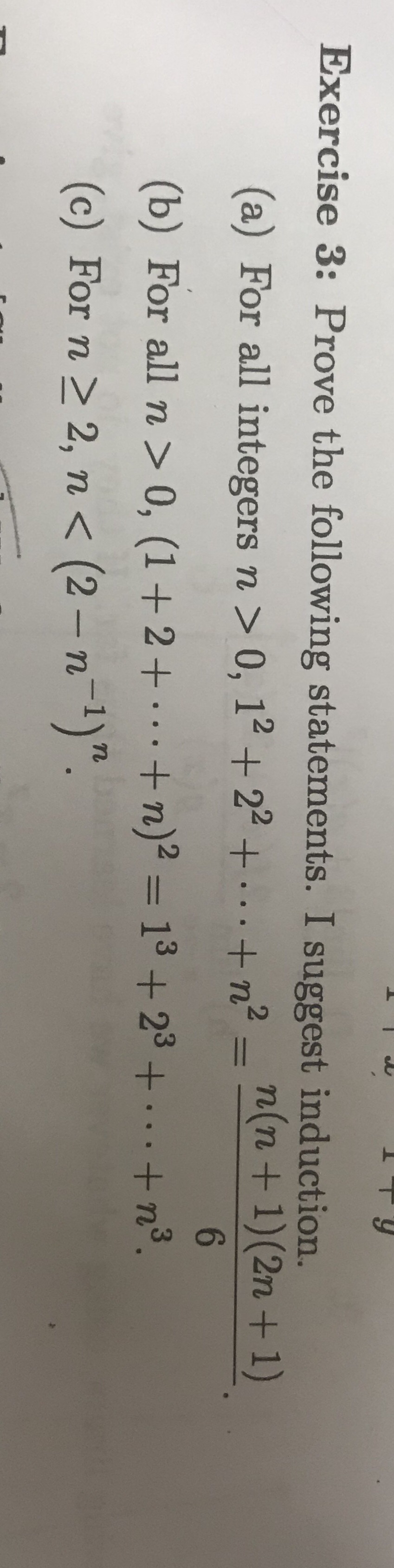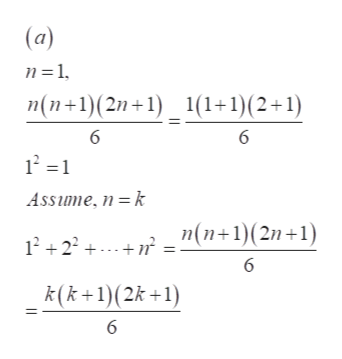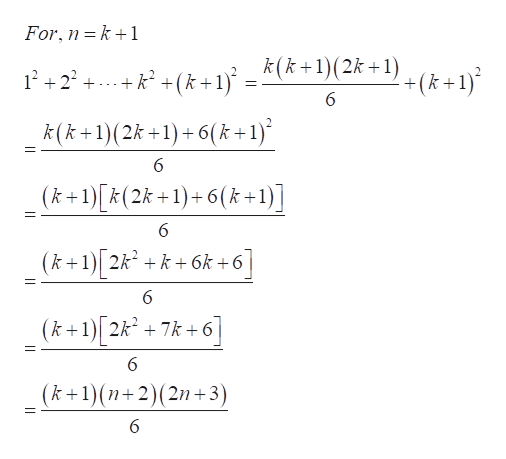# Exercise 3: Prove the following statements. I suggest induction.(a) For all integers n > 0, 12 + 22 +...+n-n(n+1) (2n + 1)6(b) For all n > 0, (1+2+.+ n)2 = 13 + 23 + .+n3.(c) For n2, n < (2 -n1)".

Question
107 views

Exercise 5 questions B and/or Chelp_outlineImage TranscriptioncloseExercise 3: Prove the following statements. I suggest induction. (a) For all integers n > 0, 12 + 22 +...+n-n(n+1) (2n + 1) 6 (b) For all n > 0, (1+2+.+ n)2 = 13 + 23 + .+n3. (c) For n 2, n < (2 -n1)". fullscreen
check_circle

Step 1

Refer to the question, in mathematical induction first prove that the equation holds for n=1.

Then we have to assume that the equation holds for n=k and then prove that the equation also holds for n=k+1. Here the provided equation in first part is,

Step 2

Now follow the steps as mentioned above.help_outlineImage Transcriptionclose(a) n 1 n(n+1)(2n+1) 1(1+1)(2+1) 6 6 121 Assime, n =k "(n+1)(2n+1) 12+22 6 k(k+1)(2k +1) | fullscreen
Step 3

Now prove for n=k+1 and then  by induction on n, the equa...help_outlineImage TranscriptioncloseFor, n k1 +k +(k+1}* _ k(k+1)(2k+)+(k +1) 122 6 k(k+1)(2k +1)6(k+1)* (k+1)[k(2k+1)+6(k+1)] 6 6k +6 (k1 2kk 6 (k+1) 2k27k+6] (k+1)(n+2)(2n+3) 6 fullscreen

### Want to see the full answer?

See Solution

#### Want to see this answer and more?

Solutions are written by subject experts who are available 24/7. Questions are typically answered within 1 hour.*

See Solution
*Response times may vary by subject and question.
Tagged in